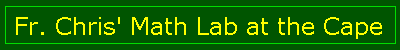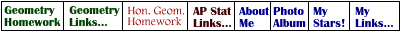Main Page

Lessons
Angles
Pool
Oil Pump
Basic Triangles
More Triangles
Art
Web Page
Euclid
Homework
Geometry
Hon Geometry

Web Calc
Pythagorean
Square Root
Fractions

Trivia
Impossibles
Trivia Quiz
Optical Quiz

TI Calc
Astronomy
Capuchins
FAQ

E-mail me!

# Medians and Altitudes of a Triangle

## Instructions

Use the Basic Triangles or the More Triangles
1. If you make an isosceles triangle ABC with the bases at A(-5, 8) and and B(-5,-10), where would C have to be so that the base angles measure 59 °?

2. Would C be closer or farther to AB if angle C increases?

3. What is the length of AC if all the angles were equal (use the co-ords of question #1)?

4. Do the medians of a triangle ever intersect at a point? Always or only for certain types of triangles?

5. Do the altitudes of a triangle ever intersect at a point? Always or only for certain types of triangles?

6. Can you find the co-ords of a triangle ABC such that is equilateral?

7. Can you find the co-ords of a triangle ABC such that it is isosceles?

8. Can you find the co-ords of a triangle such that it has a perpendicular bisector, but is neither equilateral nor isosceles?

9. What kind of triangles have altitudes outside the triangle itself?

10. Is it ever possible to have all the altitudes of a triangle outside the triangle itself?

11. Is the median ever the altitude? Always or only for certain types of triangles?

12. True or false, an angle bisector bisects the side opposite the bisected angle?

13. True or false, a median bisects the angle at the vertex from which it is drawn?

14. True or false, the length of a median is equal to half the length of the side it bisects?
Send me e-mail (cct@ktb.net)
You are visitor numbersince November 29, 1998